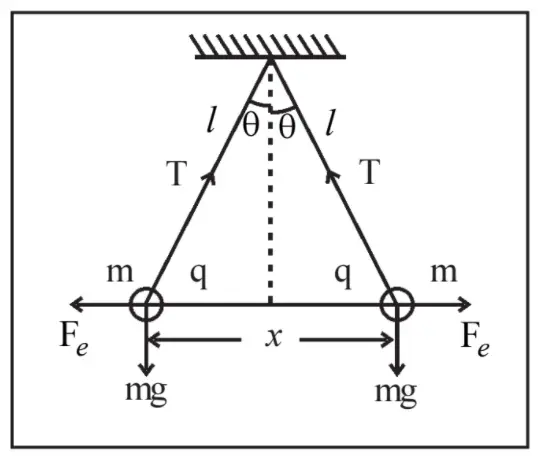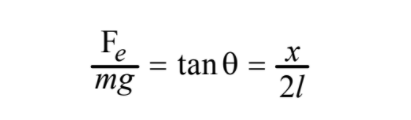# Identically Charged spheres of the same mass vertically suspended are in equilibrium – derive formulas

Say, two Identically Charged spheres of the same mass are suspended vertically and they got to be in equilibrium. Now, here we will derive the formulas related to this equilibrium condition.figure 1:Identically Charged spheres of the same mass vertically suspended are in equilibrium – derive formulas

As shown in figure 1, let the spheres be of mass m and each of these is charged with charge q. These are suspended vertically from the same point with two separate strings. Being identically charged they will repel each other, and say at the time of equilibrium the distance between the spheres be x. And say, each of the strings makes an angle of θ with the vertical line.

tan θ = (x/2)/l = x/(2l) ……………. (1)

Again, using geometry, tan θ = Fe/(mg) ………..(2) [ refer to figure 1]figure 2: equilibrium of 2 vertically suspended & identically charged balls of equal mass and related equations

Merging equation (1) and (2) we get

x/(2l) = Fe/(mg)

x = (2l) Fe/(mg) ……………. (3)
[So this is the distance between the two masses when they are in equilibrium.]

Now, Fe = q2/(4πε0)r2

Putting this expression for Fe in equation (3) we get a modified equation of x as

x = [ 2q2 l / (4πε0 mg)]1/3 …………………. (4)